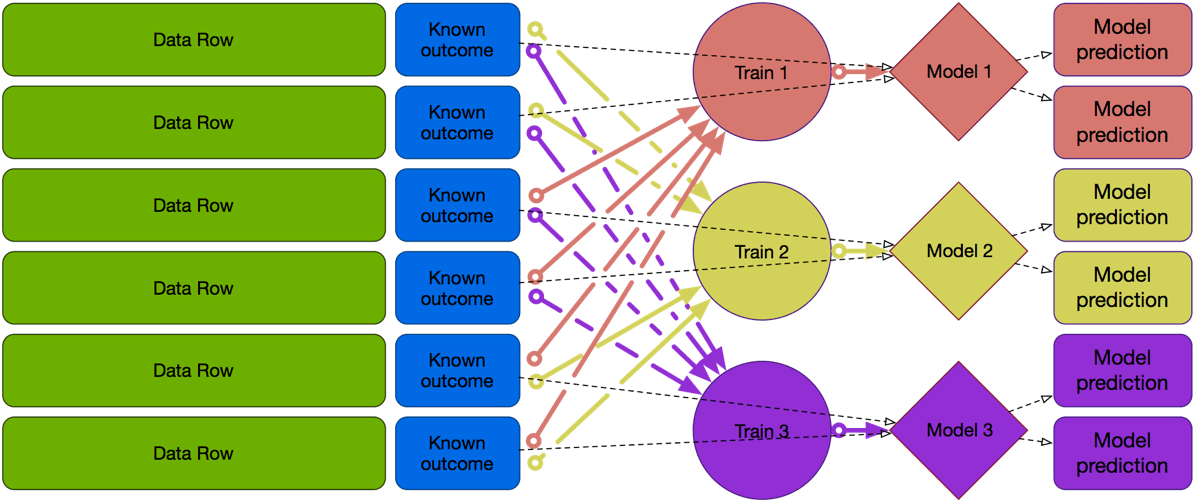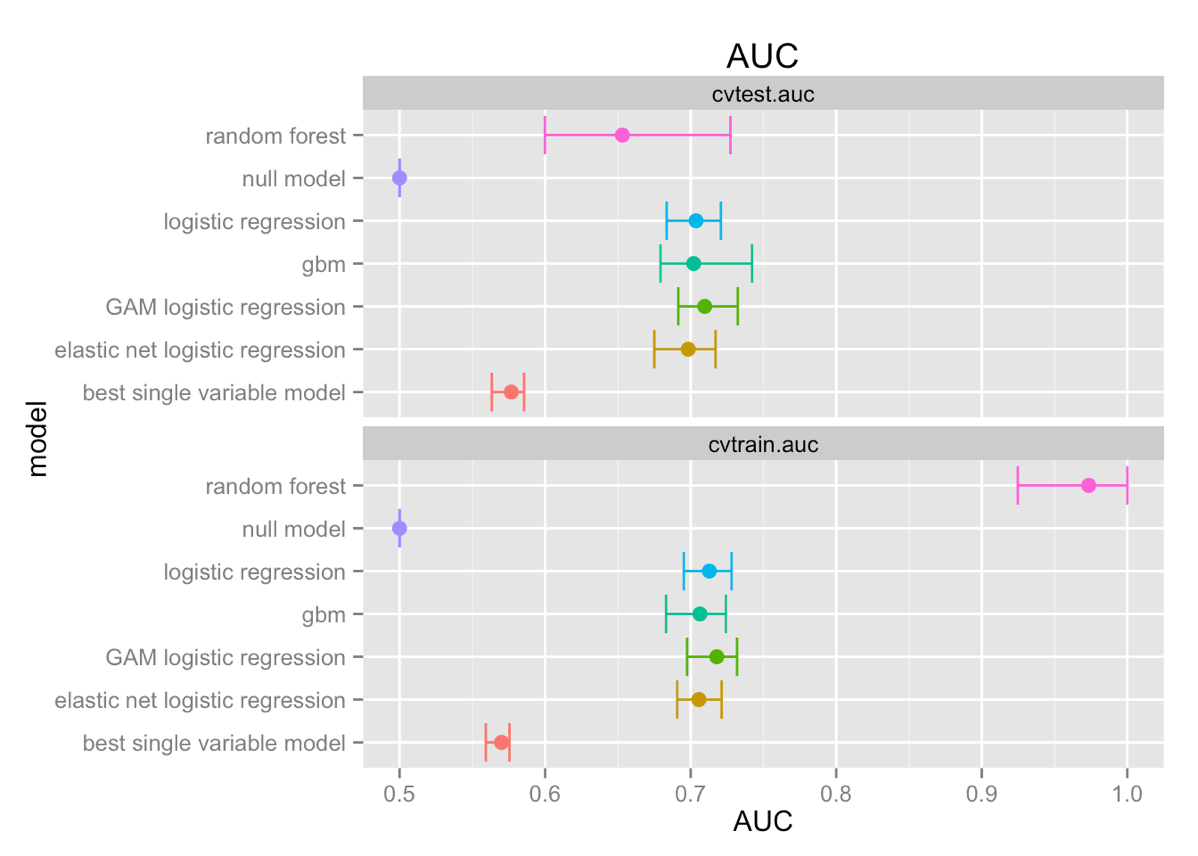# How do you know if your model is going to work? Part 4: Cross-validation techniques

Authors: John Mount (more articles) and Nina Zumel (more articles).

In this article we conclude our four part series on basic model testing.

When fitting and selecting models in a data science project, how do you know that your final model is good? And how sure are you that it’s better than the models that you rejected? In this concluding Part 4 of our four part mini-series “How do you know if your model is going to work?” we demonstrate cross-validation techniques.

Previously we worked on:

### Cross-validation techniques

Cross validation techniques attempt to improve statistical efficiency by repeatedly splitting data into train and test and re-performing model fit and model evaluation.

For example: the variation called k-fold cross-validation splits the original data into k roughly equal sized sets. To score each set we build a model on all data not in the set and then apply the model to our set. This means we build k different models (none which is our final model, which is traditionally trained on all of the data).Notional 3-fold cross validation (solid arrows are model construction/training, dashed arrows are model evaluation).

This is statistically efficient as each model is trained on a 1-1/k fraction of the data, so for k=20 we are using 95% of the data for training.

Another variation called “leave one out” (which is essentially Jackknife resampling) is very statistically efficient as each datum is scored on a unique model built using all other data. Though this is very computationally inefficient as you construct a very large number of models (except in special cases such as the PRESS statistic for linear regression).

Statisticians tend to prefer cross-validation techniques to test/train split as cross-validation techniques are more statistically efficient and can give sampling distribution style distributional estimates (instead of mere point estimates). However, remember cross validation techniques are measuring facts about the fitting procedure and not about the actual model in hand (so they are answering a different question than test/train split).

Though, there is some attraction to actually scoring the model you are going to turn in (as is done with in-sample methods, and test/train split, but not with cross-validation). The way to remember this is: bosses are essentially frequentist (they want to know their team and procedure tends to produce good models) and employees are essentially Bayesian (they want to know the actual model they are turning in is likely good; see here for how it the nature of the question you are trying to answer controls if you are in a Bayesian or Frequentist situation).

Remember cross validation is only measuring the effects of steps that are re-done during the cross validation. So any by-hand variable transformations or pruning are not measured. This is one reason you want to automate such procedures, so you can include them in the cross validated procedures and measure their effects!

For the cross validation below we used a slightly non-standard construction (code here). We then repeated five times splitting the data into calibration, train, and test; and repeated all variable encodings, pruning, and scoring steps. This differs from many of the named cross-validation routines in that we are not building a single model prediction per row, but instead going directly for the distribution of model fit performance. Due to the test/train split we still have the desirable property that no data row is ever scored using a model it was involved in the construction of.

This gives us the following graphs:In this case the error bars are just the minimum and maximum of the observed scores (no parametric confidence intervals). Again, the data suggests that one of the variants of logistic regression may be your best choice. Of particular interest is random forest, which shows large error bars. This means that random forest (on this type of data, with the variable treatment and settings that we used) has high variance compared to the other fitting methods that we tried. The random forest model that you fit is much more sensitive to the training data that you used.

For more on cross-validation methods see our free video lecture here.

## Takeaways

Model testing and validation are important parts of statistics and data science. You can only validate what you can repeat, so automated variable processing and selection is a necessity.

You can become very good at testing and validation, if instead of working from a list of tests (and there are hundreds of such tests) you work in the following way:

1. Ask: What do I need to measure (a size of effect and/or a confidence)?
2. Ask: Do I have enough data to work out of sample?
3. Ask: Am I okay with a point estimate, or do I need distributional details?
4. Ask: Do I want to measure the model I am turning in or the modeling procedure?

The answers to these questions or trade-offs between these issues determines your test procedure. That is why this series was organized a light outline of typical questions leading to traditional techniques.

This concludes our series.

• Part 1: The problem
• Part 2: In-training set measures
• Part 3: Out of sample procedures
• Part 4: Cross-validation techniques (this part)### jmount

Data Scientist and trainer at Win Vector LLC. One of the authors of Practical Data Science with R.

### 2 replies ›

1.Stephen says:

Nice series of articles. Thought provoking.

One minor issue – the link at the end for the entire series of articles in one document (http://www.win-vector.com/blog/2015/09/isyourmodelgoingtowork/) goes nowhere. Maybe cross-validation could have helped here… Ok, bad joke…

1.jmount says:

Eeek! Sorry about the bad link. I am going to share the whole article in one piece, but not until tomorrow. Thanks for the correction. I have added a note that the link isn’t valid yet.

Actually there is a funny testing story to that: for me the link works as I am administrator and can see articles before they are published.